#### You may also like### Exploring Cubic Functions

Quadratic graphs are very familiar, but what patterns can you explore with cubics?### Number Rules - OK

Can you produce convincing arguments that a selection of statements about numbers are true?### Take Three from Five

Caroline and James pick sets of five numbers. Charlie chooses three of them that add together to make a multiple of three. Can they stop him?

# Quadratic Matching

##### Age 14 to 16Challenge Level

Can you match each graph to one of the statements (so that each graph is paired with a single statement)?

(1)                                                                 (2)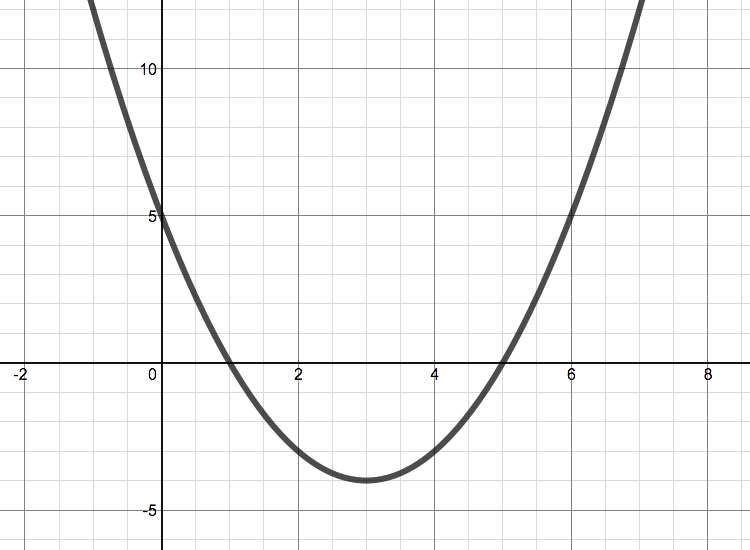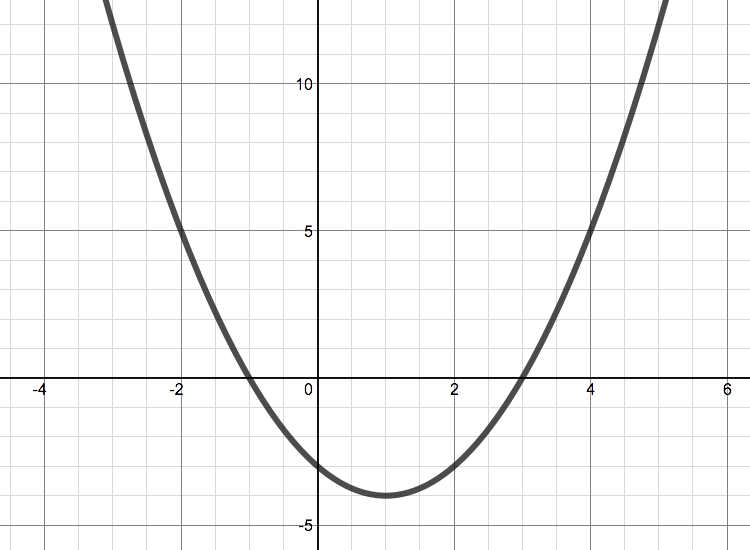(3)                                                                 (4)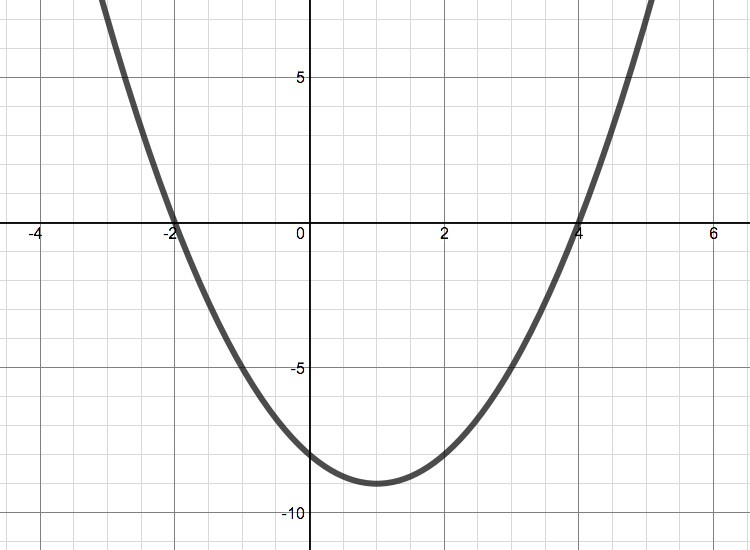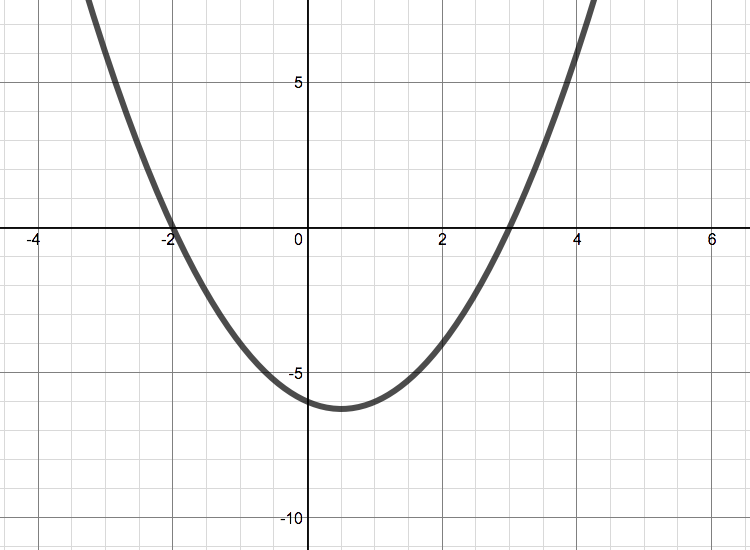(5)                                                                 (6)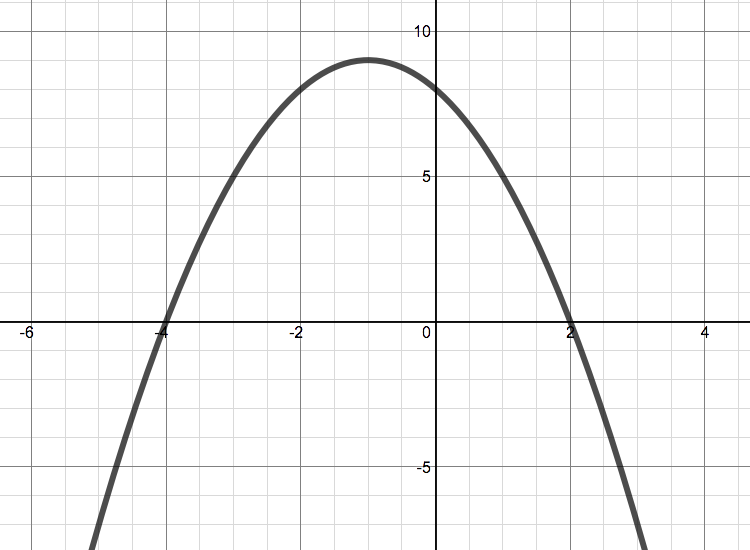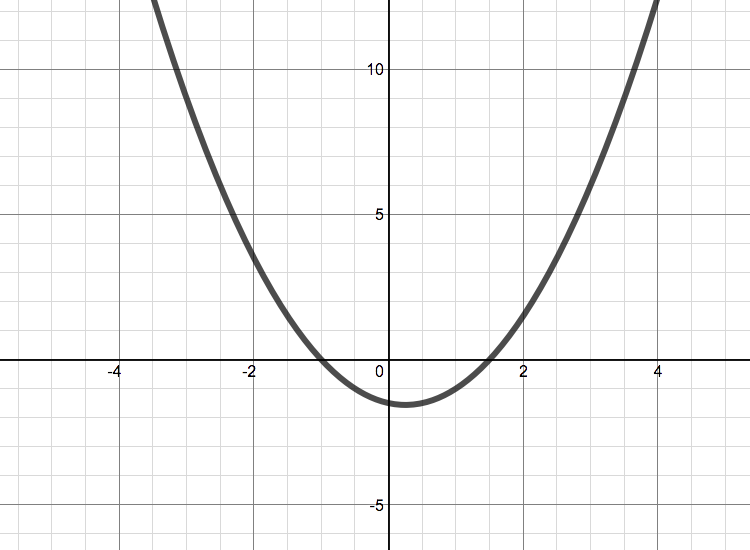(7)                                                                 (8)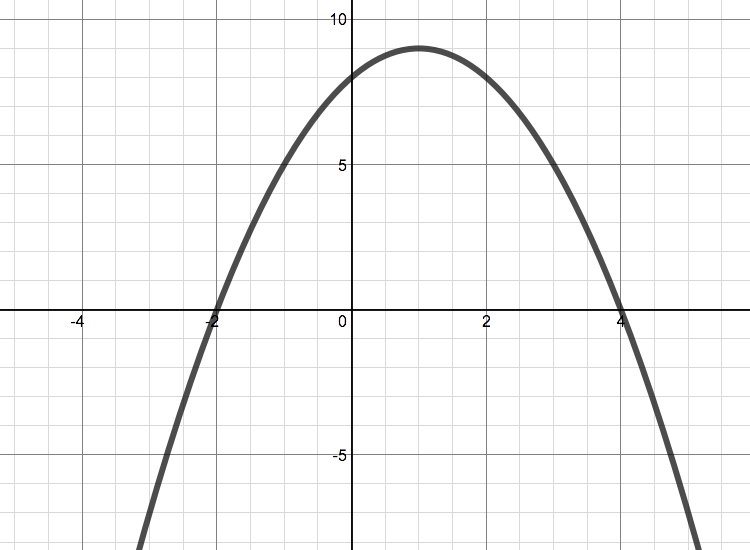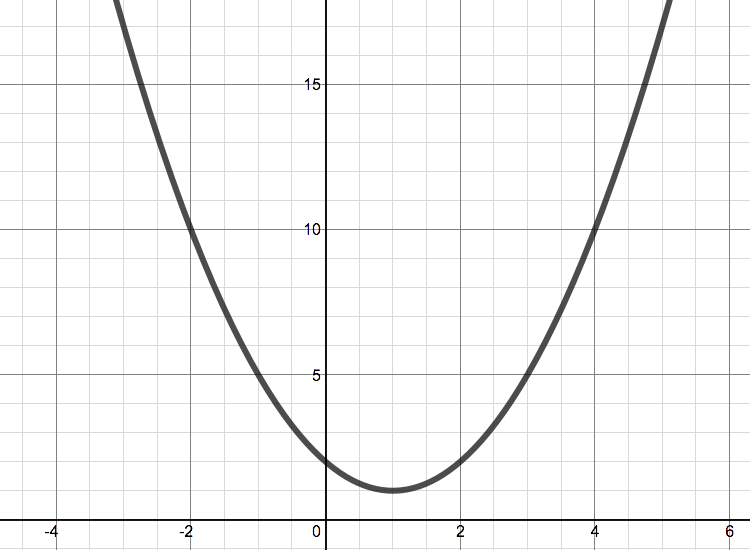(9)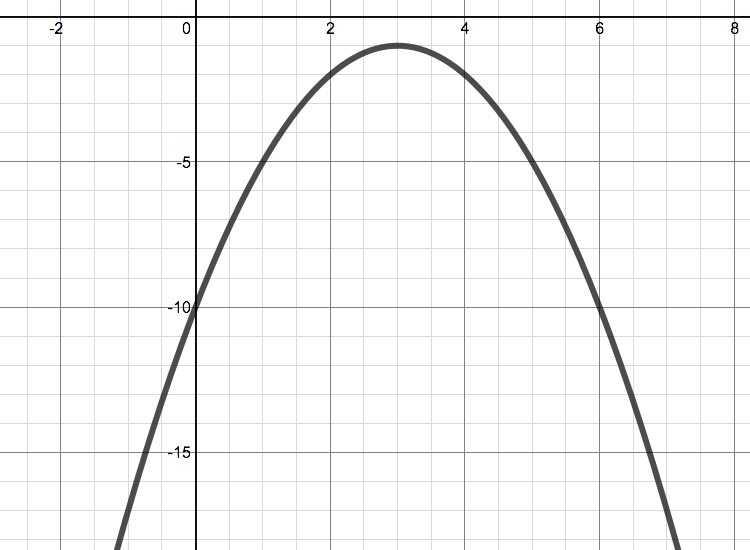Assume that all the graphs have an equation of the form $y= ax^2 + bx + c$.

(a) The line of symmetry of this graph is $x=3$.

(b) This function has a non-integer root.

(c) The line of symmetry of this graph is $x=k$, where $k<0$.

(d) The $y$ values for this graph are all greater than $0$ (that is, $y>0$).

(e) The vertex of this graph lies on the line $x=1$.

(f) The constant term of this function is $-8$ (that is, $c=-8$).

(g) The sum of the roots of this function are $6$.

(h) The points $(0,8)$ and $(2,8)$ both lie on this curve.

(i) The sum of the roots of this function is an odd number (that is, $\frac ba$ is odd).

With thanks to Don Steward, whose ideas formed the basis of this problem.